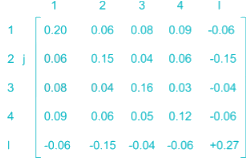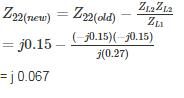Courses

# Test: Power Systems- 5

## 10 Questions MCQ Test RRB JE for Electrical Engineering | Test: Power Systems- 5

Description
This mock test of Test: Power Systems- 5 for Electrical Engineering (EE) helps you for every Electrical Engineering (EE) entrance exam. This contains 10 Multiple Choice Questions for Electrical Engineering (EE) Test: Power Systems- 5 (mcq) to study with solutions a complete question bank. The solved questions answers in this Test: Power Systems- 5 quiz give you a good mix of easy questions and tough questions. Electrical Engineering (EE) students definitely take this Test: Power Systems- 5 exercise for a better result in the exam. You can find other Test: Power Systems- 5 extra questions, long questions & short questions for Electrical Engineering (EE) on EduRev as well by searching above.
QUESTION: 1

### A power system has 200 busses including 15 generator buses. For the load flow analysis using the Newton-Raphson method in polar coordinates, the size of the Jacobian is

Solution:

Total number of buses (n) = 200

Number of generator buses = 15

One generator bus acts as slack bus.

Size of Jacobian = (2 × 200) - 2 - 14

= 384

*Answer can only contain numeric values
QUESTION: 2

### The Zbus of a system is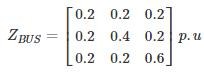If a 3 phase fault occurs at BUS – 2, the p.u. fault current in each phase is – (in pu)

Solution:

Three phase fault current is given by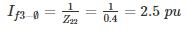QUESTION: 3

### A single line diagram of a power system is shown in the figure. The per unit values of line impedances are given. The sum of diagonal elements of YBUS matrix is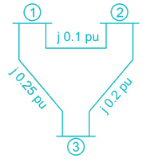Solution:

Z12 = j0.18 pu ⇒ y12 = -j10 pu

Z13 = j0.25 pu ⇒ y13 = -j4 pu

Z23 = j0.2 pu ⇒ y23 = -j5 pu

Y11 = y12 + y13 = -j14 pu

Y22 = y21 + y23 = -j15 pu

Y33 = y31 + y32 = -j9 pu

Sum of diagonal elements = -j14 – j15 – j9

= -j38 pu

*Answer can only contain numeric values
QUESTION: 4

The power system network is having a 60 bus system. The YbusYbus of this power system network is having 70% of sparse. The minimum number of transmission lines that exist in the bus system___

Solution:

Total elements in Ybus = 60 × 60 = 3600

Non – zero = 0.3 × 3600 = 1080

Diagonal = 60

Mutual elements = 660

Transmission lines =1020/2=510

QUESTION: 5

The single line diagram of a power system network having 3 buses and 4 lines is shown in the figure below. The line data is provided in the associated table. The values of the (2, 2) and (2,1) elements of the bus admittance matrix (YBUS) are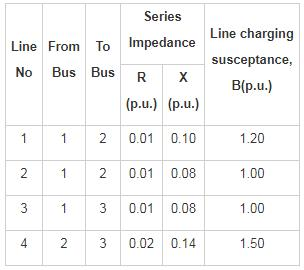Solution:

From the table

Impedance of line 1 = 0.01 + j0.01
Admittance of line 1 =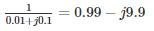Impedance of line 2 = 0.01 + j0.08

Admittance of line 2 =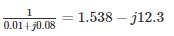Resultant admittance between the bus1 and bus2

y21 = y12 = 0.99 – j9.9 + 1.538 – j 12.3

= 2.528 – j22.2

Impedance of line 3 = 0.01 + j0.08

Admittance of line 3 =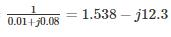y13 = 1.538 – j12.3

Impedance of line 4 = 0.02 + j0.14

Admittance of line 4 =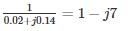Charging susceptance at bus2

2yc2 = sum of charging susceptance of line 1, 2 and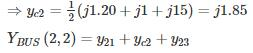= 2.528 - j22.2 + j1.85 + 1 - j7

= 3.528 – j 27.35

YBUS(2,1) = - y21

= - 2.528 + j22.2

*Answer can only contain numeric values
QUESTION: 6

For a Z bus system Z11 = j0.25,Z12 = j0.02,Z13 = j0.05,z14 = j0.04

There are 2 generators at bus 1 and 3 and their sub-transient reactance was induced while calculating Z bus. A three-phase fault occurs at bus 1. The magnitude of pu current supplied by generator 3 whose sub-transient reactance is j0.05 pu is___ (in pu)

Solution: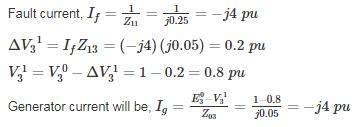QUESTION: 7

The bus admittance matrix for the network shown in the figure is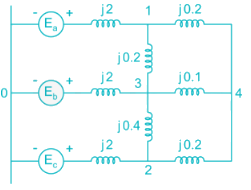Solution:

After converting the given impedances into admittances,

y11 = -j0.5 - j5 - j5 = -j10.5

y22 = -j0.5 - j2.5 - j2.5 = -j5 = -j8.0

y33 = -j0.5 - j5 - j10 - j2.5 = -j18.0

y44 = -j5 - j10 - j5 = -j20.0

y12 = y21 = 0

y13 = y31 = j5.0

y14 = y41 = j5.0

y23 = y32 = j2.5

y24 = y42 = j5.0

y34 = y43 = j10.0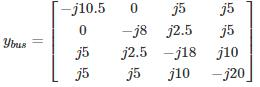QUESTION: 8

Two generators G1 and G2 are connected with cable having a reactance of j3 PU and the load demand at two buses are SD1 = 20 + j20 PU and SD2 = 25 + j2.5 PU the total reactive power in PU at the generating station G1 when δ = 20° is ______ PU

Solution: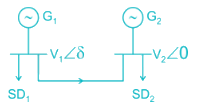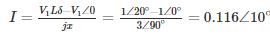S = V1I* = 1∠20° × .116∠-10 = 0.116∠10

S= SD1 + s

= 20 + 20j + .114 + 0.020j

S1 = 20.114 + 20.02 j

Total power = Active power + Reactive power

∴ Reactive power = 20.02 PU

QUESTION: 9

A generator is connected to an infinite bus through a double circuit line as shown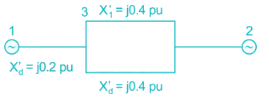The admittance matrix Y is given by

Solution: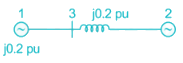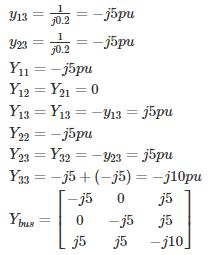QUESTION: 10

The bus impedance matrix of a 4 – bus power system network is given by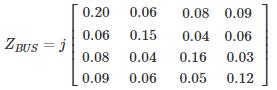An element having an impedance of j 0.12 pu is connected b/n ref bus and bus 2. Calculate new value of Z22.

Solution: